# High Level New Pattern Quantitative Aptitude Questions For IBPS Clerk Mains (Day-02) | With Video Explanation

Dear Aspirants, Quantitative Aptitude plays a crucial role in Banking and all other competitive exams. To enrich your preparation, here we have provided New Pattern Aptitude Questions for IBPS Clerk Mains. Candidates those who are going to appear in IBPS Clerk Mains can practice these questions daily and make your preparation effective.

Note: Dear IG ‘ians, Once Again IBPS Guide Team has brought you here with a New Innovative, to make you understand the questions more easier we have started providing Video Solutions for the questions. Practice here & click the link given below to check the video solutions of the same questions. Make use of it & thanks for your love.

[WpProQuiz 4579]

### Click Below for Video Solutions for these Questions

Directions (1 – 5): Study the following information carefully and answer the given questions:

The number of students who appears the exam of two different institutes (XYZ and ABCD) over the years,

2014: The number of students who appear in the exam from XYZ institute is 300 and the 60% of the students pass the examination. 30% of the students passed in the exam from ABCD institute.

2015: The number of students who appear in the exam from XYZ institute is 400 and 45% of the students pass the exam. 45% of the students pass the exam from ABCD institute.

2016: The number of students who appear in the exam from ABCD institute is 280 and 60% of the students pass the exam. 50% of the students pass the exam from XYZ institute.

2017: The number of students who appear the exam from XYZ institute and ABCD institute is 380 and 400 respectively.

1) What is the average number of passed students over the years from XYZ institute?

Statement I: If in the year 2017 the total number of students who passed from XYZ institute is 40% and the total number of students who passed from XYZ institute in 2016 is 150.

Statement II: If the ratio of the number of students passed from XYZ institute in 2017 and 2016 is 4:5.

a) Only I

b) Only II

c) Either I or II sufficient

d) All I and II necessary to the answer the question

e) The question can’t be answered even with all I and II

2) Quantity I: If the number of students who appear the exam from ABCD in 2014 is 20% less than that of the number of students who appear the exam from XYZ  institute in 2015, then what is the average number of passed students from ABCD institute in the year 2014 and 2016 together?

Quantity II: What is the difference between the total number of students who failed in the year 2015 from XYZ and the total number of students failed in the year 2016 from ABCD institute?

a) Quantity I > Quantity II

b) Quantity I ≥ Quantity II

c) Quantity II > Quantity I

d) Quantity II ≥ Quantity I

e) Quantity I = Quantity II or Relation cannot be established

3) If the number of students who appeared in the exam from XYZ  institute in the year 2018 decreased by 20% from that of students appeared in the year 2015 and 30% students failed, then what is the number of passed students from XYZ  institute in 2018 is approximately what percent more than that of the number of students passed from ABCD institute in 2016?

a) 33%

b) 37%

c) 39%

d) 31%

e) 36%

4) If the number of students who appears the exam from ABCD institute in the year 2014 is 20% more than that of the number of students who appears the exam from ABCD institute in 2017 and the number of students who appears the exam from ABCD institute in 2015 is 360. 30% of the number of students failed from ABCD institute in 2017, then find the total number of students who failed from ABCD institute in the given year?

a) 766

b) 824

c) 785

d) 815

e) None of these

5) If the number of students passed the exam from XYZ institute in 2016 is 180, the number of students who appears the exam from XYZ institute in 2016 is approximately what percent of the number of students who appears the exam from ABCD institute from 2017?

a) 90%

b) 80%

c) 92%

d) 88%

e) None of these

Directions (6 – 10): A number is given in each question with three series I, II and III. Find the series which exactly replaces the question mark with the number and choose the options accordingly.

6) 96

I. 152, 148, 132, (?), 32

II. 16, 32, (?), 384

III. 18.5, 17.5, 33, (?), 380

a) Only I

b) Only II

c) Only III

d) Both I and II

e) All I, II and III

7) 52

18, 25, (?), 128, 386

16, 20, 26, 36, (?), 76

III. 6, 14, 24, 36, (?), 66

a) Only III

b) None

c) Both I and II

d) Only II

e) All I, II and III

8) 139

I. 11178, 3726, 1242, 414, (?), 46

II. 15.5, 33, 68, (?), 278

III. 39, 41, 47, 59, 79, (?), 151

a) Only I

b) None

c) Both II and III

d) Both I and III

e) Both I and II

9) 1369

I. 1319, 1329, 1344, (?), 1404, 1459

II. 1194, 1315, 1171, 1340, 1144, (?)

III. 593, 780, 968, 1160, (?), 1640

a) Both I and II

b) Only I

c) Only III

d) None

e) All I, II and III

10) 64

I. 1, 8, 27, (?), 125, 216

II. 30, 31, (?), 195, 784

III. 32, (?), 256, 1536, 12288

a) None

b) Both I and II

c) Both II and III

d) All I, II and III

e) Neither I nor II

### Click Below for Video Solutions for these Questions

Directions(1-5):

From Statement I,

Number of students passed from XYZ in 2017=40/100*380=152

Number of students passed from XYZ in 2016=150

Number of students passed from XYZ in 2014=300*60/100=180

Number of students passed from XYZ in 2015=400*45/100=180

Average= (152+150+180+180)/4 = 662/4

So, Statement I alone is sufficient to the answer the question.

From Statement II,

If the ratio of the number of students passed from XYZ institute in 2017 and 2016 is 4:5.

So, Statement II alone is not sufficient to the answer the question.

From quantity I,

Number of students appeared from ABCD in 2014=400*80/100=320

Number of students passed from ABCD in 2014=320*30/100=96

Number of students passed from ABCD 2016=280*60/100=168

Average= (96+168)/2=132

From quantity II,

Number of students failed from XYZ 2015=400*55/100=220

Number of students failed from ABCD 2016=280*40/100=112

Difference=220-112=108

Quantity I > Quantity II

Number of students appeared from XYZ institute in 2018=400*80/100=320

Number of students passed from XYZ in 2018=320*70/100=224

Number of students passed from ABCD in 2016=280*60/100=168

Required percentage = [(224 – 168)/168]*100 = 33.33%

ABCD in 2014=400*120/100=480

ABCD in 2015=360

Number of students failed from ABCD in 2014=480*70/100=336

Number of students failed from ABCD in 2015=360*55/100=198

Number of students failed from ABCD in 2016=280*40/100=112

Number of students failed from ABCD in 2017=400*30/100=120

Required total= (336+198+112+120) = 766

Number of students passed the exam from XYZ institute in 2016=180*100/50=360

Number of students passed the exam from ABCD institute in 2017=400

Required percentage = 360*(100/400) = 90%

Directions (6-10):

I – series pattern: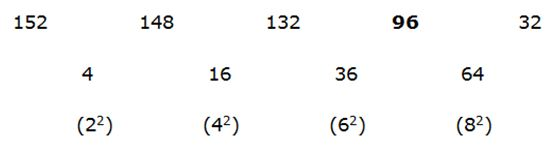II – series pattern:

16 * 2 = 32

32 * 3 = 96

96* 4 = 384

III – series pattern:

18.5 * 1 – 1 = 17.5

17.5 * 2 – 2 = 33

33 * 3 – 3 = 96

96*4 – 4 = 380

All the three given series exactly replaces the same number in the question mark.

I – series pattern:

18 * 1.5 – 2 = 25

25 * 2 + 2 = 52

52 * 2.5 – 2 = 128

128 * 3 + 2 = 386

II – series pattern: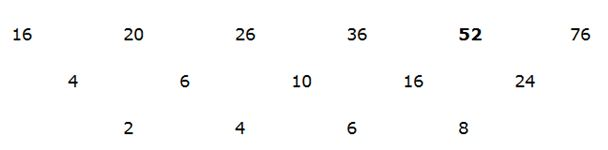III – series pattern: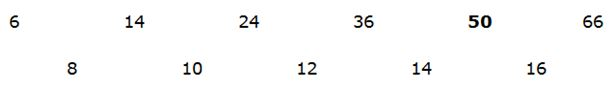In all the given series, only I and II exactly replaces the same number in the question mark.

I – series pattern:

11178/3 = 3726

3726/3 = 1242

1242/3 = 414

414/3 = 138

138/3 = 46

II – series pattern:

15.5 * 2 + 2 = 33

33 * 2 + 2 = 68

68 * 2 + 2 = 138

138 * 2 + 2 = 278

III – series pattern:

39 + (1*2) = 41

41 + (2*3) = 47

47 + (3*4) = 59

59 + (4*5) = 79

79 + (5*6) = 109

109 + (6*7) = 151

(Or)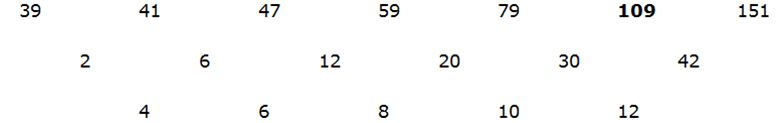In all the given series, no one exactly replaces the same number in the question mark.

I – series pattern:

1319 + (5*2) = 1329

1329 + (5*3) = 1344

1344 + (5*5) = 1369

1369 + (5*7) = 1404

1404 + (5*11) = 1459

II – series pattern:

1194 + 121 = 1315

1315 – 144 = 1171

1171 + 169 = 1340

1340 – 196 = 1144

1144 + 225 = 1369

III – series pattern: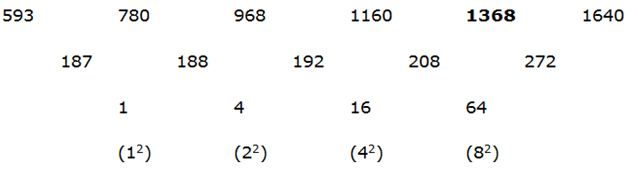In all the given series, only I and II exactly replaces the same number in the question mark.

I – series pattern:

13 = 1

23 = 8

33 = 27

43 = 64

53 = 125

63 = 216

II – series pattern:

(30 + 1)* 1 = 31

(31+1)*2 = 64

(64+1)*3 = 195

(195+1)*4 = 784

III – series pattern:

32 * 2 = 64

64 * 4 = 256

256 * 6 = 1536

1536 * 8 = 12288

All the three given series exactly replaces the same number in the question mark.